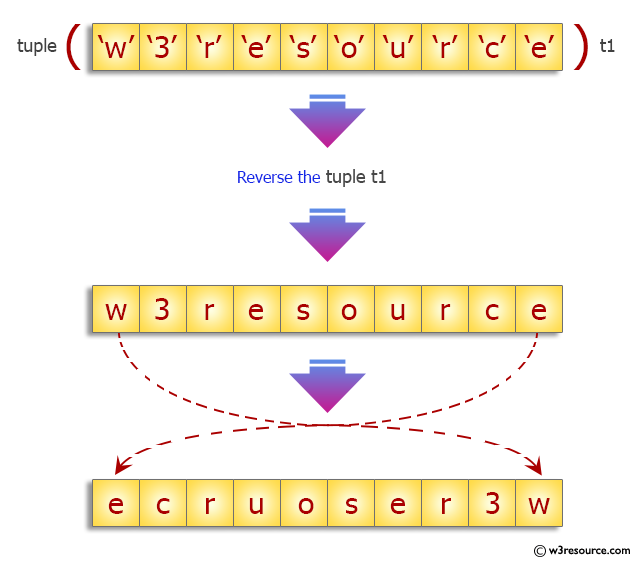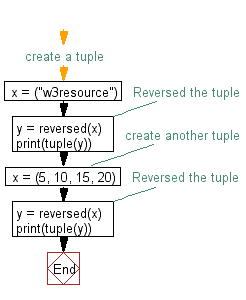﻿ Python: Reverse a tuple - w3resource# Python Exercise: Reverse a tuple

## Python tuple: Exercise-18 with Solution

Write a Python program to reverse a tuple.

Sample Solution:-

Python Code:

``````#create a tuple
x = ("w3resource")
# Reversed the tuple
y = reversed(x)
print(tuple(y))
#create another tuple
x = (5, 10, 15, 20)
# Reversed the tuple
y = reversed(x)
print(tuple(y))
```
```

Sample Output:

```('e', 'c', 'r', 'u', 'o', 's', 'e', 'r', '3', 'w')
(20, 15, 10, 5)
```

Pictorial Presentation:Flowchart:## Visualize Python code execution:

The following tool visualize what the computer is doing step-by-step as it executes the said program:

Python Code Editor:

Have another way to solve this solution? Contribute your code (and comments) through Disqus.

What is the difficulty level of this exercise?

Test your Programming skills with w3resource's quiz.

﻿

## Python: Tips of the Day

Decapitalizes the first letter of a string:

Example:

```def tips_decapitalize(s, upper_rest=False):
return s[:1].lower() + (s[1:].upper() if upper_rest else s[1:])
print(tips_decapitalize('PythonTips'))
print(tips_decapitalize('PythonTips', True))
```

Output:

```pythonTips
pYTHONTIPS
```